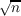#### Vol. 18, No. 1, 1966

 Download this articleFor screen For printingRecent Issues Vol. 323: 1 Vol. 322: 1  2 Vol. 321: 1  2 Vol. 320: 1  2 Vol. 319: 1  2 Vol. 318: 1  2 Vol. 317: 1  2 Vol. 316: 1  2Online Archive Volume: Issue:The Journal Subscriptions Editorial Board Officers Contacts Submission Guidelines Submission Form Policies for Authors ISSN: 1945-5844 (e-only) ISSN: 0030-8730 (print) Special Issues Author Index To Appear Other MSP Journals
A combinatorial problem in the symmetric group

### Oscar S. Rothaus and John Griggs Thompson

Vol. 18 (1966), No. 1, 175–178
##### Abstract

If G is a group and T is a nonempty subset of G, we say that T divides G if and only if G contains a subset S such that every element of G has a unique representation as ts with t in T, s in S, in which case we write T S = G. We study the case where G is Σn, the symmetric group on n symbols and T is the set consisting of the identity and all transpositions in Σn.

The problem may be given a combinatorial setting as follows: For x, y in Σn, let d(x,y) be the minimum number of transpositions needed to write xy1. One verifies that d converts Σn into a metric space, and that T divides Σn if and only if Σn can be covered by disjoint closed spheres of radius one.

We use the irreducible characters of Σn, together with judiciously selected permutation representations of Σn, to prove the following result.

Theorem. If 1 + (n(n 1))2 is divisible by a prime exceeding+ 2, then T does not divide Σn.

Primary: 20.20
##### Milestones